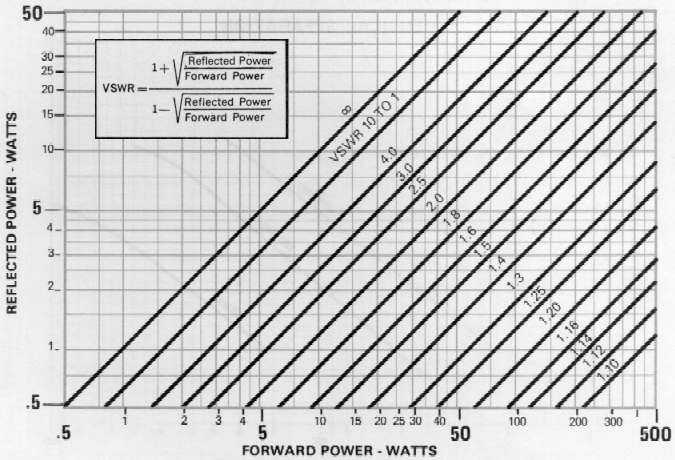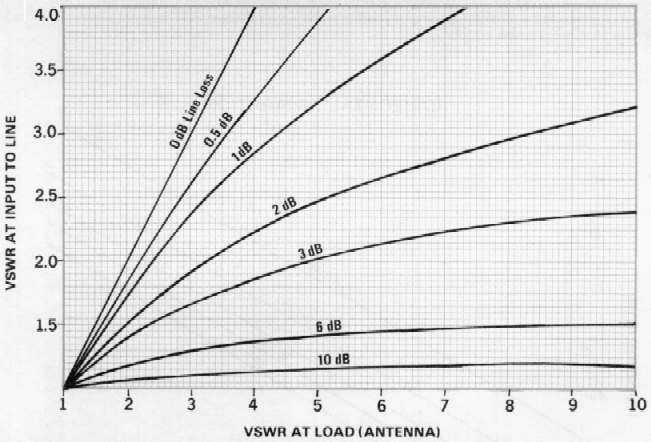VSWR MONOGRAPHVSWR CONVERSION CHARTFIELD MEASUREMENT OF VSWR: An inline watt meter will provide acceptance accuracy for measuring VSWR of antenna system. Typically, a base station antenna system is expected to have a VSWR of 1.5 to 1, or less, measured at the antenna. To determine the VSWR, measure the forward power and reverse power. Use the above VSWR Monograph to find VSWR. If the measurements of 100 watts forward power and 4 watts reflected power represents a VSWR of 1.5 to 1.

If VSWR is measured at the station end of the transmission line, the indicated VSWR will not be the actual VSWR at the antenna because of attenuation in the transmission line. The above VSWR Conversion Chart can be to determine the actual VSWR at the antenna terminals. For example, assume a length of transmission line which has a loss of 3 dB. If the watt meter at the station end of the line measures 50 watt forward power and 4 watts reflected power, the indicated VSWR is 1.8 to 1. Use of the VSWR Conversion Chart will show that actual VSWR at the antenna is 3.7 to.